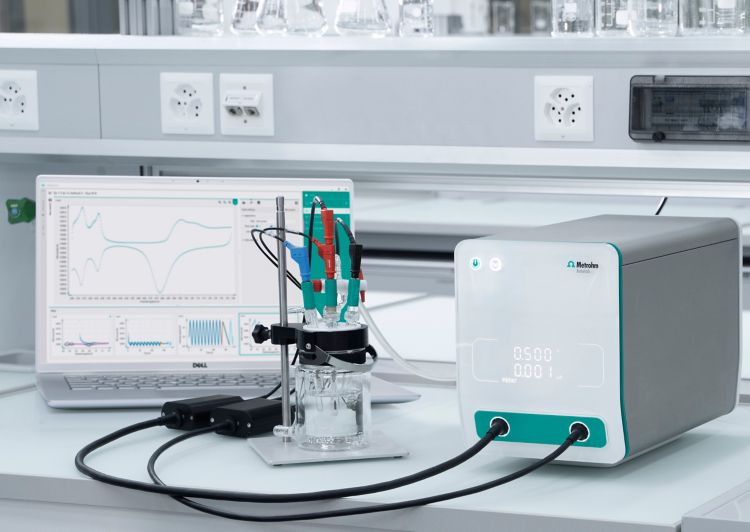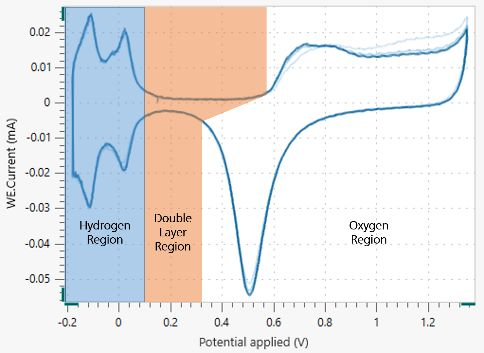The study of the electrochemical behavior of platinum in acidic media is of crucial importance in fundamental electrochemistry and electrocatalysis. Most electrocatalytic processes occurring at Pt electrodes are highly sensitive to the structure of the platinum surface.

Cyclic voltammetry (CV) is a widely used rapid measurement technique that provides both a qualitative and quantitative fingerprint of platinum surfaces. A comparison of results given by linear and staircase CVs is presented in this Application Note.The laboratory experiments in this study were performed with VIONIC powered by INTELLO from Metrohm Autolab (Figure 1).

As standard, VIONIC is equipped with a linear scan generator, allowing the possibility for users to perform linear sweeps and linear cyclic voltammetries on various samples. A Metrohm Pt wire was used as the working electrode (WE), and the counter electrode (CE) was the platinum sheet electrode. The Ag/AgCl 3 mol/L KCl electrode was used as a reference (RE).

For the electrochemical cell, an Autolab Electrocatalysis RRDE cell was employed and placed inside of a Metrohm Faraday cage.

Prior to the experiment, the glassware, electrodes, and the Teflon portion of the cell in direct contact with the electrolyte were cleaned with aggressive oxidizing solutions. A more detailed description of the cleaning protocol can be provided upon request.

An aqueous solution of sulfuric acid (0.5 mol/L H2SO4) was used as the electrolyte in this application.

Ultrapure water was used to prepare the cleaning and rinsing solutions as well as the electrolyte. The electrolyte was purged with argon gas for an hour before beginning the experiment.

The INTELLO procedure to characterize the platinum electrode surface consisted of a linear CV followed by a staircase CV. Each CV measurement began with polarizing the working electrode to 0.15 V. After a stabilization period of 3 s, the linear and staircase CV measurements followed. Each CV consisted of three scans, starting at 0.15 V, scanning up to the first vertex at 1.35 V, then scanning down to the second vertex at -0.21 V, and ultimately ending up at 0.15 V.Figure 2. A typical cyclic voltammogram of a Pt electrode in sulfuric acid with three main areas highlighted. In blue, the hydrogen region, in orange, the double layer region, and in white, the oxygen region.

In Figure 2, a typical cyclic voltammogram of a platinum electrode in acidic solution is shown, highlighting the different events that occur at the Pt-electrolyte interface.

In blue, the hydrogen adsorption-desorption region (hydrogen region) is shown. At such potentials and depending of the scan direction, hydrogen is either adsorbed or desorbed at the Pt electrode-electrolyte interface. The reaction that occurs in this region is known as the hydrogen under-potential deposition (HUPD), shown in Equation 1.

The charging and discharging of the double layer that forms the interface (known as the double layer region) is shown in orange.

In white, the oxygen region is found—the potential region where platinum is oxidized to platinum oxide during the anodic scan, and platinum oxide is reduced to platinum on the reverse scan.

In Figure 3, the linear cyclic voltammograms performed at different scan rates and step potentials www.metrohm.com/electrochemistry 3 are shown. Each color corresponds to a cycle at a different scan rate, which is explained in Table 1.

A constant sampling interval of 10 ms was kept for all measurements.

Figure 3. The linear CV performed on the platinum electrode in sulfuric acid. For the color codes, refer to Table 1.
Table 1. Relation between the colors of each cyclic voltammogram in Figure 3 and Figure 4, with the corresponding scan rates.
Data Color Scan Rate (mV/s)
Blue 500
Orange 250
Green 100
Purple 50
Black 25

In Figure 4, the staircase cyclic voltammograms performed at different scan rates and step potentials are shown. Each color corresponds to a cycle at different scan rate and step potential (Table 1). Both scan rates and step potentials were kept identical to the experiments with linear CV to have a 10 ms sampling interval time for all scans.

Figure 4. The staircase CV performed on the platinum electrode in sulfuric acid. For the color codes, refer to Table 1.

The linear cyclic voltammograms in Figure 3 exhibit peaks at negative applied potentials, assigned to the hydrogen adsorption/desorption process. Such peaks are flattened in the staircase cyclic voltammograms shown in Figure 4.

This behavior is highlighted in Figure 5, where the cyclic voltammograms recorded linearly at 500 mV/s using linear (in blue) and staircase cyclic voltammetry (in orange) are compared.

Figure 5. The cyclic voltammogram recorded at 500 mV/s using linear CV (blue) and staircase CV (orange).

Hydrogen adsorption/desorption is a fast, surface-limited highly capacitive process and occurs at time scales similar to the charging of the double layer. In the case of staircase CV, due to the «staircase» nature of the applied potential profile, the very rapidly occurring charging currents which result for each step applied in the staircase scan will not be sampled. This would lead to a much lower and erroneous current recorded for the hydrogen adsorption-desorption region. A more detailed explanation of the difference between linear and staircase scans is found in the Metrohm Application Note AN-EC-007.

In this Application Note, a comparison between linear and staircase cyclic voltammetry on a Pt electrode in sulfuric acid is given.

A visual observation of the cyclic voltammograms shows the necessity of linear CV using a linear scan in order to obtain a reliable fingerprint of the hydrogen region on platinum electrodes.

The same conclusion is also valid for many other fast electron transfers happening at catalysts immobilized at the surface of an electrode.